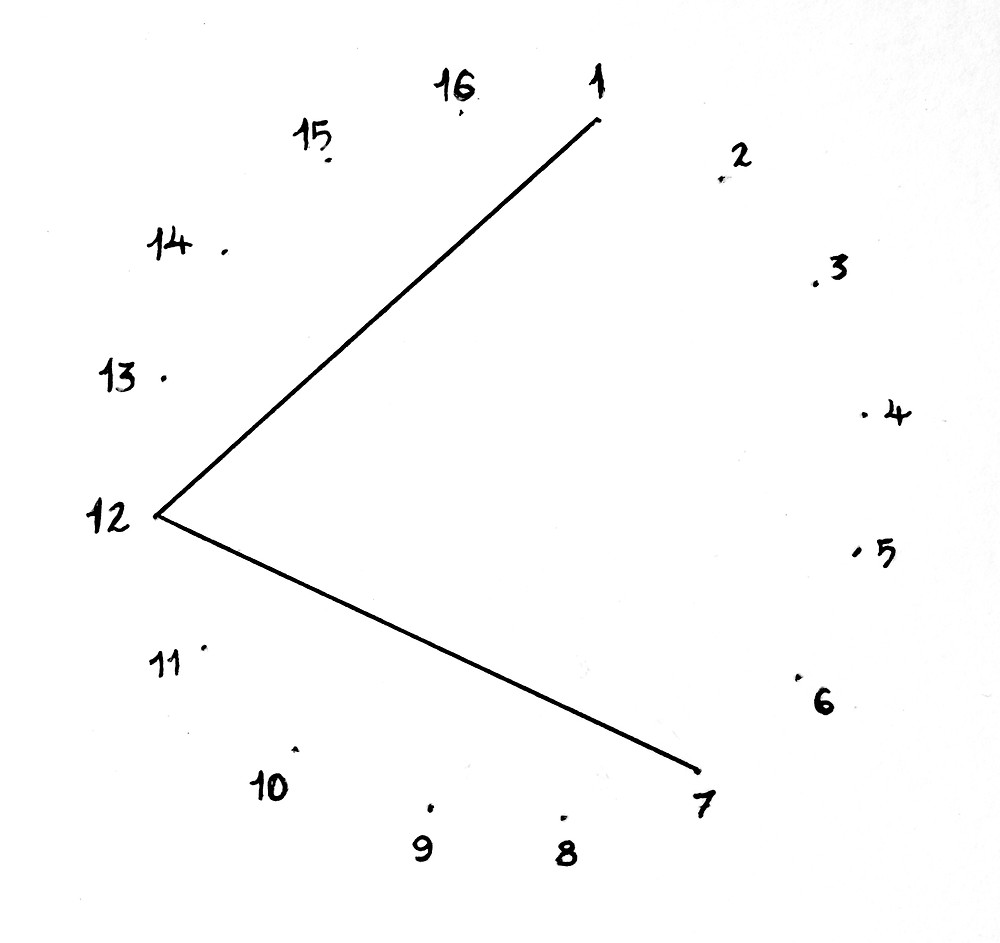Search

# How to Graph Magic Squares as Circles

Let's start with a quick, kinda awkward time lapse of me graphing an order 4, "most perfect" magic square from India!"Jaina Magic Square"

This is an ancient magic square found in the Parshvanatha temple, in Madhya Pradesh, India. It's of the class "most perfect" which means that all the broken pan-diagonal lines sum to the magic constant, in this case 34. Any group of 4 cells in the matrix will also sum to 34. Every row, column, diagonal, pan-diagonal, even the 4 corner cells sum to the magic constant in most perfect magic squares.

To graph this magic square we divide 360/16; 360 for each degree of a circle and 16 for each cell in the matrix. The number representation doesn't matter all that much when transcribing the square as a circular graph because it's a closed system at that point. The distance between 1 & 16 in the graph is equal to the distance between 1-2. You can use this same method to graph "irregular magic squares" as well, which do not utilize sequential numbers.360/16 = 22.5

360 divided by 16 equals 22.5, so all points are 22.5 degrees apart. Number them in order, in whichever direction you choose. Here I started in the top left cell of the magic square (which is #7), but the number/cell you begin the graph with does not change the resulting geometry.

Connect 7 to 12, then connect 12 to 1, 1 to 14 and finally 14 back to 7 completes the first horizontal row. Following the same steps with each horizontal row yields the graph shown below.I like to graph the sets of a square separately the first time, to be able to appreciate their unique qualities and symmetry. Graphing the vertical set is the exact same method, connecting instead 7 to 2, 2 to 16, then 16 to 9 and finally 9 connects back to 7 etc. The intersection of planes in the vertical set of this magic square is so, so beautiful to me...It's one of my absolute favorites.And now the pan-diagonals! Much like the rows and columns looped back to their originating cell/number, graphing the pan-diagonal rows requires us to imagine the entire magic square does this in every direction.The color coordinated lines in the image above illustrate the 1st pan-diagonal set, to graph it yourself imagine the same concept in the opposite direction. When graphed, the pan-diagonal sets of this magic square are mirror images of one another.1st Pan-diagonal Set © 2019 TL2nd Pan-diagonal Set © 2019 TL

If you graph all of these sets together as one, your result is:Jaina Magic Square Graph © 2019 TL

TA-DA!

You can now graph any magic as a circle!